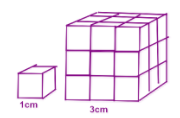# Calculate Sides and Volumes of Similar Shapes Using Volume Scale Factors

In this worksheet, students will identify similar shapes and use knowledge of proportions to work out the volume.Key stage:  KS 3

Curriculum topic:   Geometry and Measures

Curriculum subtopic:   Solve Problems Involving Perimeters and Areas of 2D Shapes

Difficulty level:#### Worksheet Overview

In this activity, we are going to be looking at the volume scale factor of similar shapesA similar shape has the same size angles, but the sides are proportionally bigger or smaller.

We use scale factor to help us to work out any missing lengths.

Area scale factor - this is where we square the scale factor.

For volume scale factor - we cube the scale factor

This might seem obvious since:

length is measured in cm
area in cm2
volume in cm3If scale factor = 2

Area scale factor is 22 = 2 x 2 = 4

Volume scale factor = 23 = 2 x 2 x 2 = 8

Let's look at a question to help us to understand this.

Example

Two similar shapes have a scale factor of 3

What is the volume scale factor?

As we said above, we can cube the scale factor to get the volume scale factor!Volume scale factor = 33 = 3 x 3 x 3 = 27

You can see from the diagram above that the volume of the bigger shape is 27 if the scale factor is 3.

Are you ready to have a go at some questions?### What is EdPlace?

We're your National Curriculum aligned online education content provider helping each child succeed in English, maths and science from year 1 to GCSE. With an EdPlace account you’ll be able to track and measure progress, helping each child achieve their best. We build confidence and attainment by personalising each child’s learning at a level that suits them.

Get started